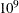# The Quadratic Programming Solver

### Problem Statistics

Optimizers can encounter difficulty when solving poorly formulated models. Information about data magnitude provides a simple gauge to determine how well a model is formulated. For example, a model whose constraint matrix contains one very large entry (on the order of) can cause difficulty when the remaining entries are single-digit numbers. The PRINTLEVEL=2 option in the OPTMODEL procedure causes the ODS table ProblemStatistics to be generated when the QP solver is called. This table provides basic data magnitude information that enables you to improve the formulation of your models.

The example output in Figure 9.4 demonstrates the contents of the ODS table ProblemStatistics.

Figure 9.4: ODS Table ProblemStatistics

The OPTMODEL Procedure

Problem Statistics
Number of Constraint Matrix Nonzeros 4
Maximum Constraint Matrix Coefficient 2
Minimum Constraint Matrix Coefficient 1
Average Constraint Matrix Coefficient 1.25

Number of Linear Objective Nonzeros 2
Maximum Linear Objective Coefficient 3
Minimum Linear Objective Coefficient 2
Average Linear Objective Coefficient 2.5

Number of Lower Triangular Hessian Nonzeros 1
Number of Diagonal Hessian Nonzeros 2
Maximum Hessian Coefficient 20
Minimum Hessian Coefficient 2
Average Hessian Coefficient 6.75

Number of RHS Nonzeros 2
Maximum RHS 100
Minimum RHS 1
Average RHS 50.5

Maximum Number of Nonzeros per Column 2
Minimum Number of Nonzeros per Column 2
Average Number of Nonzeros per Column 2

Maximum Number of Nonzeros per Row 2
Minimum Number of Nonzeros per Row 2
Average Number of Nonzeros per Row 2